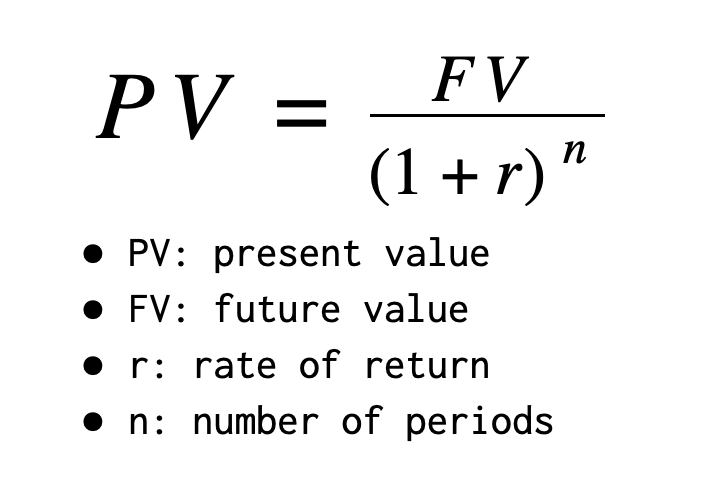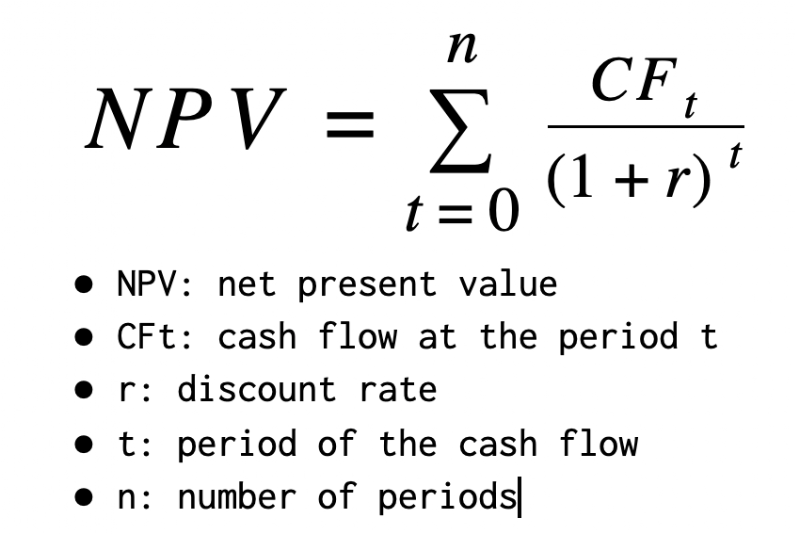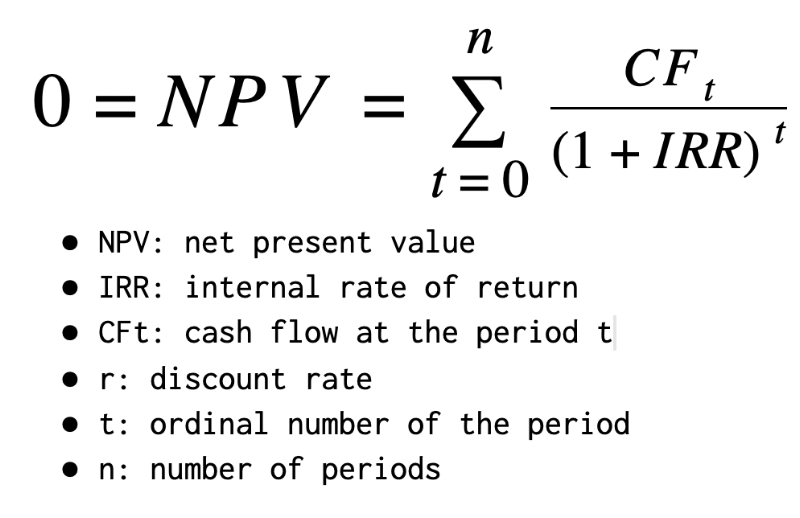### Time value of money, Present Value (PV), Future Value (FV), Net Present Value (NPV), Internal Rate of Return (IRR)

Why do I use my current money to invest in the stock market? Because I expect to have more money in the future. Why do I need more money in the future than now? Because of many reasons, the same amount of money will have less purchasing power than today. Therefore my investment needs to generate more money than today to protect my purchasing power in the future. That is the main concept of the time value of money where one dollar today is worth more than one dollar in the future.## Present Value (PV), Future Value (FV)

At 10% annual growth rate, an investment of 1000\$ will be worth `1000 * 110% = 1100\$` after 1 year, and will be worth `1000 * 110% * 110% = 1210\$` after 2 years.

• The future value of 1000\$ after 2 years at the rate of 10% is 1210\$.
• Inversely, the "present value" of 1210\$ 2 years ago at the rate of 10% is 1000\$.## Net Present Value (NPV)

Let's say I have 1000\$ now and a bank offers me a saving account at a 10% annual rate. At the same time, a public company attracts my attention because it pays a very good amount of dividend each year and has potential growth in the future. The scenario is that I would buy 100 shares at the cost of 10\$ each now and hold them for 5 years. Each year I would receive a fairly good and stable amount of dividends, at least 5% yield. After 5 years, I would sell all shares with the expectation that the share price would grow 20%. The table below shows the scenario of cash flows if I buy stocks of that company. Which investment should I choose?

YearAmountType
1\$50.00Dividend
2\$55.00Dividend
3\$60.00Dividend
4\$65.00Dividend
5\$1,200.00Sell

To make that decision, let put it this way: I want to have the same scenario of cash flows as investing into the stock market but by putting money into a saving account at a 10% annual rate. How much money do I need to put into that saving account now? Let's do the math:

• At a 10% rate, to have 50\$ after 1 year, I need to invest 45.45\$ now. Because 50\$ is the future value of 45.45\$ after 1 year at a 10% rate.
• At a 10% rate, to have 55\$ after 2 years, I need to invest 45.45\$ now. Because 55\$ is the future value of 45.45\$ after 2 years at a 10% rate.
• At a 10% rate, to have 60\$ after 3 years, I need to invest 45.08\$ now. Because 60\$ is the future value of 45.08\$ after 3 years at a 10% rate.
• At a 10% rate, to have 65\$ after 4 years, I need to invest 44.40\$ now. Because 65\$ is the future value of 44.40\$ after 4 years at a 10% rate.
• At a 10% rate, to have 1200\$ after 5 years, I need to invest 745.11\$ now. Because 1200\$ is the future value of 745.11\$ after 5 years at a 10% rate.

Totally, to have the same scenario of cash flows as investing in the stock market, I need to invest `45.45 + 45.45 + 45.08 + 44.40 + 745.11 = 925.49\$` now in a saving account at a 10% rate. In other words, to have the same result in the future, investing in the stock market costs me 1000\$ now, whereas investing in the saving account at a 10% rate costs me only 925.49\$ now. Therefore, in this case, I should better put money in the saving account at 10% rate.

Let's suppose that the share prices would grow 50% during the 5 years, which means the last cash flow would be 1500\$ in the 5th year. In this case, at a 10% rate, to have 1500\$ after 5 years, I need to invest 931.38\$ now. Because 1500\$ is the future value of 931.38\$ after 5 years at a 10% rate. Totally, to have the same scenario of cash flows as investing in the stock market, I need to invest `45.45 + 45.45 + 45.08 + 44.40 + 931.38 = 1089.88\$` now in a saving account at a 10% rate. In other words, to have the same result in the future, investing in the stock market costs me only 1000\$ now, whereas investing into the saving account at a 10% rate costs me 1089.88\$ now. Therefore, in this case, I have a better deal to invest in the stock market than to put money in a saving account at a 10% rate.

Moreover, because at year 0 future value equals to present value, I can do like that:

• For the first scenario, sum of all present values of future cash flows is: `-1000 + 45.45 + 45.45 + 45.08 + 44.40 + 745.11 = -96.40 < 0`
• For the second scenario, sum of all present values of future cash flows is: `-1000 + 45.45 + 45.45 + 45.08 + 44.40 + 931.38 = 89.88 > 0`

What's I have calculated so far is to sum the present values of future cash flows at a defined discount rate and compare it with 0. That's what they call Net Present Value (NPV). If Net Present Value (NPV) is positive, the investment is worth pursuing.## Discount rate

In this example, I have chosen the saving account at a 10% annual rate as a benchmark to evaluate my investment in the stock market. The 10% annual rate of that saving account is therefore the discount rate in my evaluation. My investment in the stock market must beat 10% annually, otherwise, it is not worth my time and effort because I can easily save that money at 10% annually. The choice of a discount rate is important and depends on personal preferences. Here are a few examples:

• A minimum required rate of return for an investment that one sets for herself/himself
• An expected rate of return if investing in an alternative asset such as: saving account, real estate, buying a business, etc.
• A reference rate of return of the market: S&P 500, CAC 40, etc.

## Internal Rate of Return (IRR)

The discount rate that makes Net Present Value (NPV) equal to zero is called the Internal Rate of Return (IRR).• For the first scenario of cash flows above, that internal rate of return is 8.13%.
• At a 8.13% rate, sum of all present values of future cash flows is: `-1000 + 46.24 + 47.04 + 47.46 + 47.54 + 811.72 = 0`.
• Because 8.13% < 10%, it confirms once again that the saving account at a 10% rate is a better choice.

YearAmountTypePresent Value
1\$50.00Dividend\$46.24
2\$55.00Dividend\$47.04
3\$60.00Dividend\$47.46
4\$65.00Dividend\$47.54
5\$1,200.00Sell\$811.72
• For the second scenario of cash flows above, that internal rate of return is 12.57%.
• At a 12.57% rate, sum of all present values of future cash flows is: `-1000 + 44.42 + 43.40 + 42.06 + 40.47 + 829.66 = 0`.
• Because 12.57% > 10%, it confirms once again that investing in the stock market is a better choice.
YearAmountTypePresent Value
1\$50.00Dividend\$44.42
2\$55.00Dividend\$43.40
3\$60.00Dividend\$42.06
4\$65.00Dividend\$40.47
5\$1,500.00Sell\$829.66

## Conclusion

In summary, Net Present Value (NPV) and Internal Rate of Return (IRR) are two methods that help me to evaluate the performance of an investment.

To evaluate an investment with Net Present Value (NPV), I follow the steps below:

• Identify all cash flows
• Pick a discount rate
• Calculate Net Present Value (NPV) by summing all present values of those cash flows
• If Net Present Value (NPV) is positive, the investment is worth pursuing

To evaluate an investment with Internal Rate of Return (IRR), I follow the steps below:

• Identify all cash flows
• Pick a discount rate
• Calculate the Internal Rate of Return (IRR) rate that makes Net Present Value (NPV) equal to 0
• If Internal Rate of Return (IRR) is bigger than the discount rate, the investment is worth pursuing

Performing those steps requires many calculations, and I don't perform them manually. I have leveraged the built-in functions of Google Sheets to do those tasks. In the next posts, I will explain how to calculate Net Present Value (NPV) and Internal Rate of Return (IRR) in Google Sheets, particularly in the context of a stock portfolio.

## Disclaimer

The post is only for informational purposes and not for trading purposes or financial advice.

## Feedback

If you have any feedback, question, or request please:

## Support this blog

If you value my work, please support me with as little as a cup of coffee! I appreciate it. Thank you!

If you read it this far, I hope you have enjoyed the content of this post. If you like it, share it with your friends!

### Use SPARKLINE column chart to create price chart with reference price

I own and follow several stocks in my investment portfolio. I pick a reference price for each stock. To effectively track the movement of a stock, I need to visualize its 52-week prices based on the reference price that I determined. In this post, I explain how to do so with the SPARKLINE column chart in Google Sheets.

### Use SPARKLINE to create 52-week range price indicator chart for stocks in Google Sheets

The 52-week range price indicator chart shows the relative position of the current price compared to the 52-week low and the 52-week high price. It visualizes whether the current price is closer to the 52-week low or the 52-week high price. In this post, I explain how to create a 52-week range price indicator chart for stocks by using the SPARKLINE function and the GOOGLEFINANCE function in Google Sheets.

### Compute cost basis of stocks with FIFO method in Google Sheets

After selling a portion of my holdings in a stock, the cost basis for the remain shares of that stock in my portfolio is not simply the sum of all transactions. When selling, I need to decide which shares I want to sell. One of the most common accounting methods is FIFO (first in, first out), meaning that the shares I bought earliest will be the shares I sell first. As you might already know, I use Google Sheets extensively to manage my stock portfolio investment, but, at the moment of writing this post, I find that Google Sheets does not provide a built-in formula for FIFO. Luckily, with lots of effort, I succeeded in building my own FIFO solution in Google Sheets, and I want to share it on this blog. In this post, I explain how to implement FIFO method in Google Sheets to compute cost basis in stocks investing.

### Slice array in Google Sheets

Many functions in Google Sheets return an array as the result. However, I find that there is a lack of built-in support functions in Google Sheets when working with an array. For example, the GOOGLEFINANCE function can return the historical prices of a stock as a table of two columns and the first-row being headers Date and Close. How can I ignore the headers or remove the headers from the results?

### How to convert column index into letters with Google Apps Script

Although Google Sheets does not provide a ready-to-use function that takes a column index as an input and returns corresponding letters as output, we can still do the task by leveraging other built-in functions ADDRESS , REGEXEXTRACT , INDEX , SPLIT as shown in the post . However, in form of a formula, that solution is not applicable for scripting with Google Apps Script. In this post, we look at how to write a utility function with Google Apps Script that converts column index into corresponding letters.

Anyone using Google Sheets to manage stock portfolio investment must know how to use the GOOGLEFINANCE function to fetch historical prices of stocks. As I have used it extensively to manage my stock portfolio investment in Google Sheets , I have learned several best practices for using the GOOGLEFINANCE function that I would like to share in this post.

### Create personal stock portfolio tracker with Google Sheets and Google Data Studio

I have been investing in the stock market for a while. I was looking for a software tool that could help me better manage my portfolio, but, could not find one that satisfied my needs. One day, I discovered that the Google Sheets application has a built-in function called GOOGLEFINANCE which fetches current or historical prices of stocks into spreadsheets. So I thought it is totally possible to build my own personal portfolio tracker with Google Sheets. I can register my transactions in a sheet and use the pivot table, built-in functions such as GOOGLEFINANCE, and Apps Script to automate the computation for daily evolutions of my portfolio as well as the current position for each stock in my portfolio. I then drew some sort of charts within the spreadsheet to have some visual ideas of my portfolio. However, I quickly found it inconvenient to have the charts overlapped the table and to switch back and forth among sheets in the spreadsheet. That's when I came to know the existen

### Create a dividend income tracker with Google Sheets by simply using pivot tables

As my investment strategy is to buy stocks that pay regular and stable dividends during a long-term period, I need to monitor my dividends income by stocks, by months, and by years, so that I can answer quickly and exactly the following questions: How much dividend did I receive on a given month and a given year? How much dividend did I receive for a given stock in a given year? Have a given stock's annual dividend per share kept increasing gradually over years? Have a given stock's annual dividend yield been stable over years? In this post, I explain how to create a dividend tracker for a stock investment portfolio with Google Sheets by simply using pivot tables.

### How to copy data in Google Sheets as HTML table

I often need to extract some sample data in Google Sheets and present it in my blog as an HTML table. However, when copying a selected range in Google Sheets and paste it outside the Google Sheets, I only get plain text. In this post, I explain how to copy data in Google Sheets as an HTML table by writing a small Apps Script program.

### Manage Stock Transactions With Google Sheets

The first task of building a stock portfolio tracker is to design a solution to register transactions. A transaction is an event when change happens to a stock portfolio, for instance, selling shares of a company, depositing money, or receiving dividends. Transactions are essential inputs to a stock portfolio tracker and it is important to keep track of transactions to make good decisions in investment. In this post, I will explain step by step how to keep track of stock transactions with Google Sheets.